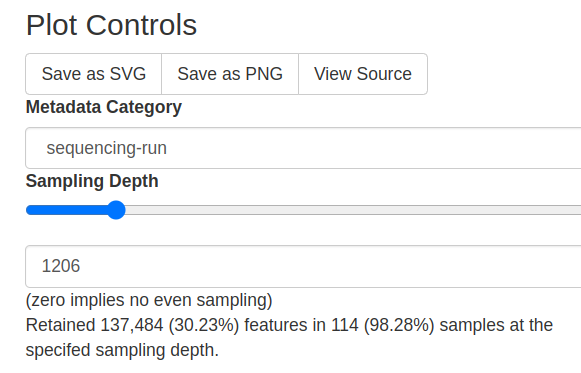# What's formula of The Retained Features number?

Hi, I'm a new QIIME2 user. For more understanding of the Feature Table, I extracted the draw data (feature-table.biom file) come from summarize step. And try to summarize by myself in Python to comprehend how Retained Features are calculated.

The input file:
data.csv (228.4 KB)
The summary file:
ftable.qzv (468.4 KB)Everything is smooth at the beginning.

## Frequency per sample

### Code

``````import pandas as pd
def countFeature(lista):
count = 0
for ele in lista:
if ele > 0:
count += 1
return count

FixList = lambda lista: [i for l in lista for i in l]

CountData = lambda features, table_data: [countFeature(table_data[table_data.index==feature].transpose().astype('float64').values) for feature in features]
SumData = lambda features, table_data: [sum(FixList(table_data[table_data.index==feature].transpose().astype('float64').values)) for feature in features]

def MakeTable(features, table_data):
count_values = CountData(features, table_data)
sum_values = SumData(features, table_data)

frePerFeature = pd.DataFrame(zip(sum_values, count_values), index=features, columns=['Frequency', 'Count'])
# print(frePerFeature)
return frePerFeature

frePerSample = pd.DataFrame(zip(sum_values, count_values), index=headers, columns=['Frequency', 'Count'])
print(frePerSample)
print(frePerSample.describe())
print(frePerSample.median())
``````

### Output:

``````                                      Frequency  Count
0bb5e24a.aa34.48e7.b1f9.e761ac2dc6b4     6141.0     89
101c02ce.4f8d.4394.83a3.0c406831d934     6473.0     83
104e5902.1c3e.417f.bb22.124bfca61a61     7040.0     85
11c4be47.fdce.4135.8acf.0894f2da5ede     2949.0     41
...                                         ...    ...
ea68ac1a.53e3.4523.924f.32ca1d2ac988     3921.0     30
eb4ece61.6afe.4c88.8a06.d616dab1dd34     2193.0     77
fa4e5dcc.7b0a.4fb1.989d.b19aec98e047     4847.0     69
fa6fda2b.4a99.4231.9325.0d74d8ae451c     4168.0     72

[116 rows x 2 columns]

Freequency       Count
count   116.000000  116.000000
mean   3921.198276   63.741379
std    1752.358208   20.189158
min     876.000000   16.000000
25%    2601.250000   51.000000
50%    3758.000000   64.500000
75%    4832.250000   77.000000
max    8273.000000  102.000000
Freequency    3758.0
Count           64.5
dtype: float64
``````

## Frequency per Features

### Code

``````features = table_data.index
frePerFeature = MakeTable(features, table_data)
print(frePerFeature)
print(frePerFeature.describe())
print(frePerFeature.median())
``````

### Output

``````                                  Frequency  Count
002e78333d6cf2b11aa7a5ba03dd2c68       59.0      3
0046913ae6f9e12dbd889671ed26c09d        5.0      1
009c3d1fd56cf5682c875f959d9fee33       63.0      4
00c7ff8350c1f1874014b473b1890bf3       17.0      1
014ed0286a2651609cc4a145b7ea6788       71.0      1
...                                     ...    ...
fe05bf03bd86129ba77552ce64c521a0        9.0      1
fe7577ac70fe20955ccd2fb1a3847a59        7.0      1
fe89f7b053b400e066cbe98313774360       16.0      3
fee475b024cb0ce7531b21360168e790        9.0      1

Frequency       Count
count    788.000000  788.000000
mean     577.232234    9.383249
std     3654.757368   17.151550
min        2.000000    1.000000
25%        8.000000    1.000000
50%       35.500000    2.000000
75%      229.750000    9.000000
max    93838.000000  103.000000
Frequency    35.5
Count         2.0
dtype: float64
``````

The above stuff corresponds Overview tab and these are correct. But when calculating the Retained Features I failed. In my opinion, the Retained Features number is the sum of the Frequency of the remaining sample. But the result is wrong. If someone elucidates me, I'll very much appreciate it.

## Interactive Sample detail

### Code

``````sample_depth = 1206

retain_sample = frePerSample[frePerSample['Frequency']>=sample_depth]

num_retain_sample = len(retain_sample)
print(f'The number of retained samples: {num_retain_sample}')

retained_num = retain_sample['Frequency'].sum()
print(f'The number of retained features: {num_retain_sample}')

``````

### Output

``````The number of retained samples:  114                    # Correct
The number of retained features: 452833.0             # Wrong
``````

I have just found out my answer. Because after the subsampling step, all sample's frequencies will equal each other and equal the Sample Depth, so the right final part is:

## Interactive

### Code

``````sample_depth = 1206

retain_sample = frePerSample[frePerSample['Frequency']>=sample_depth]
num_retain_sample = len(retain_sample)
print(f'The number of samples remain: {num_retain_sample}')
print(f'The number of samples remain: {sample_depth*num_retain_sample}')
``````

### Output

``````The number of samples remain: 114
The number of samples remain: 137484
``````
1 Like

Hi and welcome to the forum!
Glad that you already found answer at your question. And thank you for sharing the solution with the community! It can help other users as well.

1 Like

## Complement

This graph shows the dependence of the Percentage of Retained Samples and Retained Features according to the Sample Depth.

### Code

``````num_sample = len(frePerSample)
num_feature = len(frePerFeature)
max_depth = max(frePerSample['Frequency'])
total_fre = sum(frePerSample['Frequency'])

def SampleDepth(sample_depth, frePerSample):
retain_sample = frePerSample[frePerSample['Frequency']>=sample_depth]
num_retain_sample = len(retain_sample)*100/num_sample
num_retain_feature = sample_depth*num_retain_sample*100/total_fre
return num_retain_feature, num_retain_sample

import numpy as np

depths = list(range(int(max_depth)+1))

retain_feature = []
retain_sample = []
for depth in depths:
num1, num2 = SampleDepth(depth, frePerSample)
# print(num1, num2)
retain_feature.append(num1)
retain_sample.append(num2)

import matplotlib.pyplot as plt
plt.figure(figsize=(15,8))
plt.plot(depths, retain_feature, label='Percent of features');
plt.plot(depths, retain_sample, color='y', label='Percent of samples');
plt.xlabel('Sample Depth')
plt.xticks(list(range(0,int(max_depth)+1,1000)))
plt.ylabel('Percentage')
plt.ylim([0,100])
plt.xlim([0,int(max_depth)+1])
plt.legend()
plt.show()
``````
2 Likes